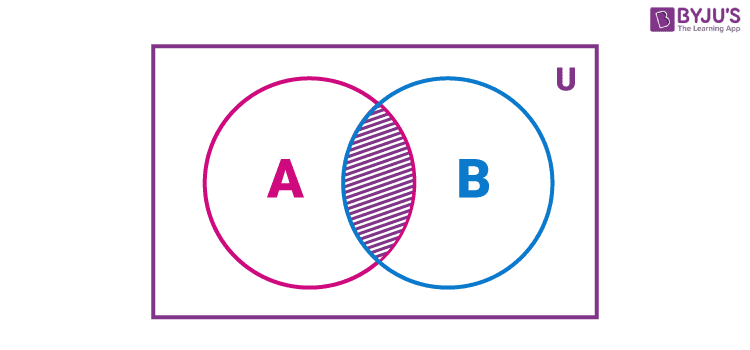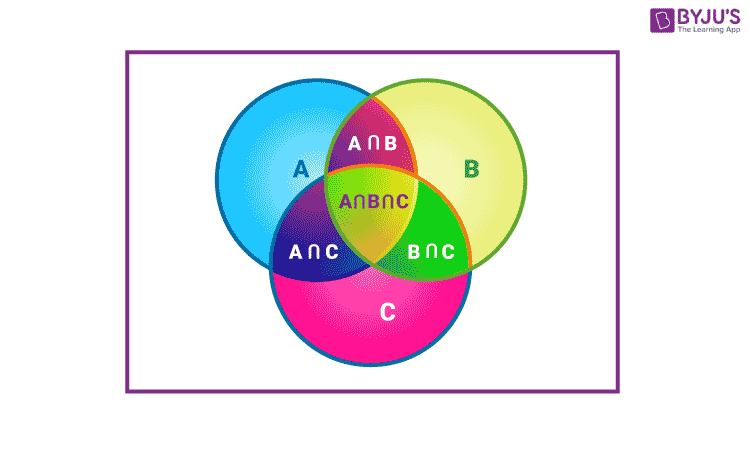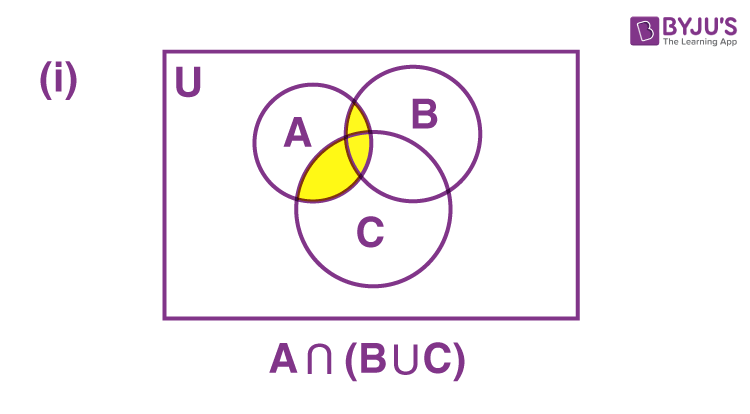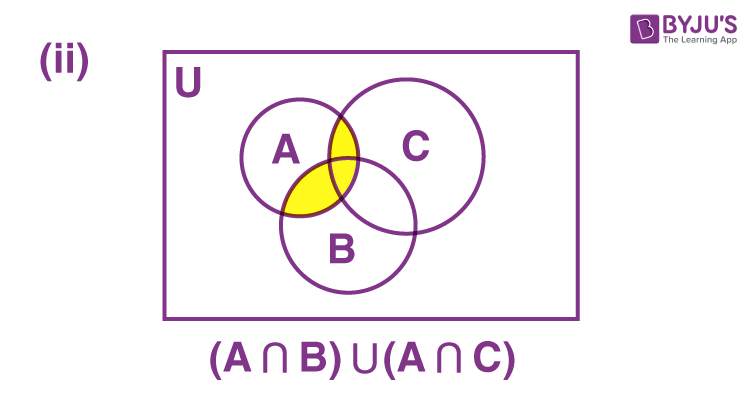# Intersection of Sets

In Mathematics, a set is a well-defined collection of objects. Unlike numbers, we can define different operations on sets and examine their properties. In set theory, an operation is an exercise to combine different sets such that a new set will be obtained with distinct properties. In this article, you will learn the intersection of sets definition, formula, expressions, along with properties and examples in detail.

## What is Intersection of Sets?

The intersection of sets A and B is the set of all elements which are common to both A and B.

Suppose A is the set of even numbers less than 10 and B is the set of the first five multiples of 4, then the intersection of these two can be identified as given below:

A = {2, 4, 6, 8}

B = {4, 8, 12, 16, 20}

The elements common to A and B are 4 and 8.

Therefore, the set of elements in the intersection A and B = {4, 8}

## Intersection of Sets Symbol

The intersection of sets can be denoted using the symbol ‘∩’. As defined above, the intersection of two sets A and B is the set of all those elements which are common to both A and B. Symbolically, we can represent the intersection of A and B as A ∩ B.

### A intersection B Formula

The formula for the intersection of sets can be given based on the definition. That means, the intersection of two sets A and B is the set containing elements that are common to both A and B. This can be expressed as:

A ∩ B = {x : x ∈ A and x ∈ B}

That means x is an element of A ∩ B, if and only if x is an element of both A and B. Thus, we can use the word “AND” to represent the intersection of sets. Sometimes, the above expression can also be referred to as the intersection of sets formula.

## Intersection of Two Sets

The intersection of two given sets, say X and Y, is the most extensive set containing all the elements common to X and Y. The intersection of two sets can be a set again with at least one element or it can be an empty set, i.e. no elements in the intersection set. Suppose A and B are two sets such that A ∩ B = φ, then A and B are called disjoint sets. That means, there are no elements at the intersection of A and B.

## Intersection of Three Sets

It is possible to find the intersection of more than two sets. In this section, you will learn how to find the intersection of three sets. Suppose A, B and C are three sets, then the intersection of these three sets is the set of all elements that are common to A, B and C. This can be represented as A ∩ B ∩ C. This can be better understood with the help of the example given below.

For example, A = {6, 8, 10, 12, 14, 16}, B = {9, 12, 15, 18, 21, 24} and C = {4, 8, 12, 16, 20, 24, 28}.

The intersection of these 3 sets can be expressed as, A ∩ B ∩ C.

Let us identify the common elements of the given sets.

12 is the only common element in A, B and C.

Therefore, A ∩ B ∩ C = {12}

### Intersection of Sets Using Venn Diagram

The intersection of two sets can be represented using the Venn diagram as shown below.In the above diagram, the shaded portion represents the intersection of two sets A and B.

Similarly, we can draw a Venn diagram for the intersection of 3 sets as shown below.In the above diagram, we can see that the centermost region denotes the intersection of three sets A, B and C.

### Intersection of Sets Properties

Some Properties of the Operation of Intersection are listed below:

(i) Commutative law: A ∩ B = B ∩ A

Consider two sets A = {1, 2, 3, 4, 5, 6} and B = {2, 3, 5, 7}.

Now, A ∩ B = {1, 2, 3, 4, 5, 6} ∩ {2, 3, 5, 7} = {2, 3, 5}

B ∩ A = {2, 3, 5, 7} ∩ {1, 2, 3, 4, 5, 6} = {2, 3, 5}

Therefore, A ∩ B = B ∩ A.

(ii) Associative law: (A ∩ B) ∩ C = A ∩ (B ∩ C)

Let A = {1, 2, 3, 4}, B = {3, 4, 5, 6}, and C = {5, 6, 7, 8 }.

Now,

A ∩ B = {1, 2, 3, 4} ∩ {3, 4, 5, 6} = {3, 4}

(A ∩ B) ∩ C = {3, 4} ∩ {5, 6, 7, 8} = { } = φ

And

B ∩ C = {3, 4, 5, 6} ∩ {5, 6, 7, 8} = {5, 6}

A ∩ (B ∩ C) = {1, 2, 3, 4} ∩ {5, 6} = { } = φ

Therefore, (A ∩ B) ∩ C = A ∩ (B ∩ C)

(iii) Law of φ and U: φ ∩ A = φ, U ∩ A = A

Consider φ = { } and A = {10, 11, 12}.

φ ∩ A = { } ∩ {10, 11, 12} = { } = φ

Let U = {2, 4, 6, 8, 10, 12, 14, 16, 18, 20} and A = {4, 8, 12, 16, 20}.

U ∩ A = {2, 4, 6, 8, 10, 12, 14, 16, 18, 20} ∩ {4, 8, 12, 16, 20} = {4, 8, 12, 16, 20} = A

(iv) Idempotent law: A ∩ A = A

Suppose A = {a, b, c, d, e} such that A ∩ A = {a, b, c, d, e} ∩ {a, b, c, d, e} = {a, b, c, d, e} = A

(v) Distributive law: A ∩ (B U C) = (A ∩ B) U (A ∩ C), i. e., ∩ distributes over U

Let us take three sets A = = {2, 4, 6, 8}, B = {2, 3, 5, 7} and C = {3, 4, 5, 6}.

B U C = {2, 3, 5, 7} U {3, 4, 5, 6} = {2, 3, 4, 5, 6, 7}

A ∩ (B U C) = {2, 4, 6, 8} ∩ {2, 3, 4, 5, 6, 7} = {2, 4, 6}

A ∩ B = {2, 4, 6, 8} ∩ {2, 3, 5, 7} = {2}

A ∩ C = {2, 4, 6, 8} ∩ {3, 4, 5, 6} = {4, 6}

(A ∩ B) U (A ∩ C) = {2} U {4, 6} = {2, 4, 6}

Therefore, A ∩ (B U C) = (A ∩ B) U (A ∩ C)

## Intersection of Sets Examples

Go through the examples given below to get a thorough understanding of the concept.

Example 1: If A = { 3, 5, 7, 9, 11}, B = {7, 9, 11, 13}, and C = {11, 13, 15}, then find B ∩ C and A ∩ B ∩ C.

Solution:

Given,

A = { 3, 5, 7, 9, 11}

B = {7, 9, 11, 13}

C = {11, 13, 15}

B ∩ C = {11, 13}

A ∩ B ∩ C = {11}

Example 2: Represent the following using Venn diagrams.

(i) A ∩ (B ∪ C)

(ii) (A ∩ B) ∪ (A ∩ C)

Solution:

Venn diagram of A ∩ (B ∪ C)Venn diagram of (A ∩ B) ∪ (A ∩ C)### Union and Intersection of Sets

The union of two sets A and B is the set of all those elements which are either in A or in B, whereas the intersection of two sets A and B is the set of all elements which are common. We can define the formulas for both union and intersection of given sets based on the cardinality of sets as given below.

If A and B are finite sets such that A ∩ B = φ, then

n (A ∪ B) = n (A) + n (B).

If A ∩ B ≠ φ, then

n (A ∪ B) = n (A) + n (B) – n (A ∩ B)

### Practice Problems

1. If P = {1, 2, 3, 4}, Q = {3, 4, 5, 6}, find P Q.
2. If A = {1, 2, 3, 4}, B = {3, 4, 5, 6}, C = {5, 6, 7, 8 }and D = { 7, 8, 9, 10 }, then calculate the following:
(i) A ∩ B
(ii) B ∩ C
(iii) A ∩ C ∩ D
(iv) A ∩ C
(v) B ∩ D
(vi) A ∩ (B ∪ C)
1. Consider three sets A = {1, 2, 3}, B = {2, 3, 5} and C = {4, 5, 6}. Find (A ∩ B) ∩ C.

## Video Lesson on What are Sets## Frequently Asked Questions on Intersection of Sets

### What is the intersection of a set?

The intersection operation on sets gives the set of elements that are common in the given sets.

### What is ∩ called?

The symbol ∩ denotes the intersection of sets. For example, the intersection of two sets X and Y can be represented as X ∩ Y.

### What is union and intersection of sets?

The union of two sets A and B is the set of all those elements which are either in A or in B, i.e. A ∪ B, whereas the intersection of two sets A and B is the set of all elements which are common. The intersection of these two sets is denoted by A ∩ B.

### What is another word for intersection?

The other word for intersection is AND which means we can represent the intersection of A, B as A and B or A ∩ B.

### Is A ∩ B is equal to B ∩ A?

Yes, A ∩ B is equal to B ∩ A. This represents the commutative law of the intersection of sets.